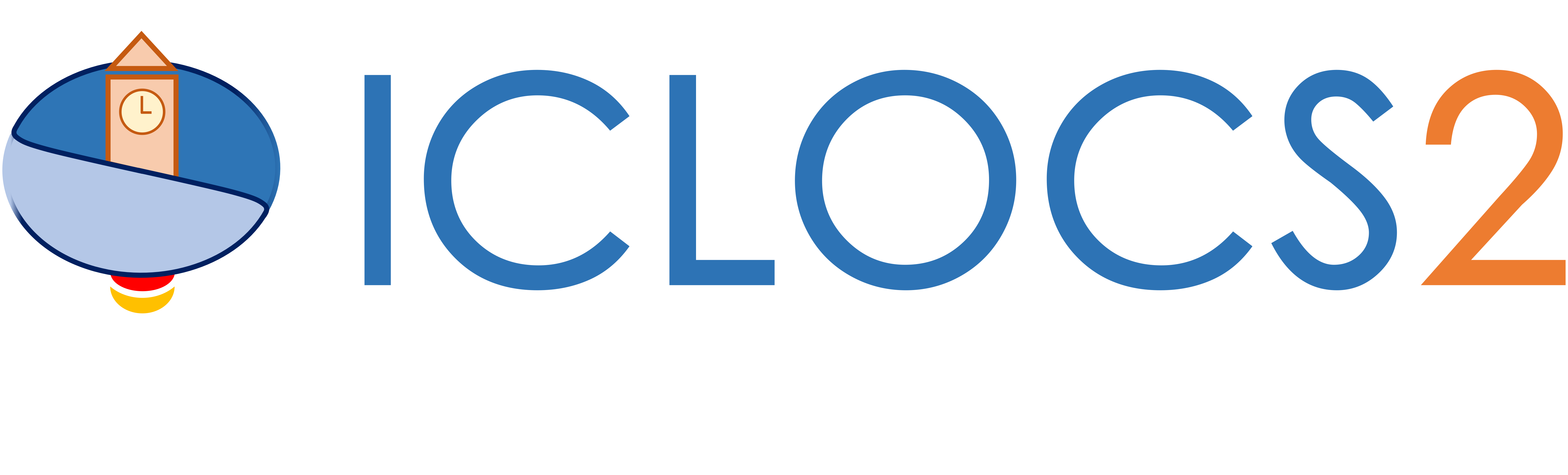Get Started

Solving a single phase problem

This guide will help you setup the solve of a single phase optimal control problem with main.m.

Fetch, transcribe and solve the problem

First we load the formulated optimal control problem (in myProblem.m)

[problem,guess]=myProblem;

Then we can load the corrsponding options and solver settings. For fixed order h methods, use

options= problem.settings(Nps);

with the input argument Nps being the number of equally spaced mesh points, unless the mesh has been specifically specified in the options. For pseudo spectral methods, two input arguments are needed. The first option is to have

options= problem.settings(Nps,Npd);

with scalar numbers used for Nps equally spaced intervals with the same polynoimial orders of Npd. For example, options= problem.settings(5,4) means using 5 LGR intervals each of polynomial degree of 4.

Alternatively, you can supply two arrays in the argument with customized meshing. For example, settings([-1 0.3 0.4 1],[4 5 3]) will have 3 segments on the normalized interval [-1 0.3], [0.3 0.4] and [0.4 1], with polynomial order of 4, 5, and 3 respectively.

Next, the problem can be transcribed and solved using the following standard command

[solution,MRHistory]=solveMyProblem( problem,guess,options);

with the returning structure variable solution containing the raw output from the NLP solver (sampled data points), the represented continous time solution and outcomes of error analysis.

Optional functions

Generation of figures

In ICLOCS 2.5, various figures can be generated with a single line of command

genSolutionPlots(options, solution);

The type of plots to be generated is spcified in the settings file with options.plot having the values

• 1: Plot all figures (state and input trajectory, multipliers/costate values and errors)
• 2: Plot only the state and input trajectory
• 3: Plot only the multipliers/costate values
• 4: Plot only the error values (absolute local error, relative local error and absolute constraint violation error)

Open-loop simulation

After obtaining the optimal control solution, it is often useful to check the implementation of the control trajectory through an open-loop simulation. This can be done by ODE integration of a simulation model that may or may not be the same as the internal model used for solving the OCP. The result of the simulation can provide insights in terms of solution quality (discretization errors), and how sensitive the optimal solution will be in case of uncertainties due to disturbances, model mismatches or simply just numerial integration errors. This will have the benefit, for example, to identify solutions that requires closed-loop implementations.

With ICLOCS 2.5, open-loop simulation of the obtained solution can be simply obtained by

[ tv, xv, uv ] = simulateSolution( problem, solution);

with tv, xv and uv the simulation output for the time, states and input variables respectively. Additionally, you many specify a preferred Matlab ODE solver with

[ tv, xv, uv ] = simulateSolution( problem, solution, 'ode113');

or

[ tv, xv, uv ] = simulateSolution( problem, solution, 'ode113', 'ode113', 0.01 );

which also specify the integration time step.

It is also possible that the state and input variables for the internal model are different from that of the real plant (the simulation model). For example in the car parking problem, the actual car have steering angle as control input; however for the internal model, steering rate is implemented as a control variable instead in order to pose a rate constraint on it. To properly simulate this, we used the following command for simulation

[ tv, xv, uv ] = simulateSolution( problem, solution, 'ode113', 0.01,[5;2]);

to allow the trajectory of the fifth state variable (steering angle) of the optimal control solution to be considered as the second input variable (steering angle) of the simulation dynamics.

Solution variables

Here we provide short descriptions for some of the main information contained in the solution variable

• multipliers: a structure variable containing the multiplier/costate information
• computation_time: time spent on solving the NLP problem
• z: the NLP variable vector returned from the NLP solver
• t0: the initial time
• tf: the terminal(final) time
• p: parameters values (part of optimization solution)
• X: sampled state values at mesh points
• x0: initial states
• U: sampled input/control values at mesh points
• T: a time array corresponding to the mesh points
• z_orgscale: the NLP variable vector after transforming back to the original variable scale
• multipliers: a structure variable containing information at all collocation points (for Hermite-Simpson method)
• Xp: Constructed continuous state trajectory
• dXp: Constructed continuous state rate (dynamics) trajectory
• Up: Constructed input/control trajectory
• Error: the absolute local error for state variables
• ErrorRelative: the relative local error for state variables
• ConstraintError: the absolute constraint violation error (organized as [path constraint upper bound, path constraint lower bound, state upper bound, state lower bound, input upper bound, input lower bound]
• T_ConstraintError: a time array corresponding to the absolute constraint violation error evaluation
• NumActiveConstraint: the number of active constraints
• TSeg_Bar: time interval barriers between LGR polynomial segments (p/hp method only)
• dX: sampled state rate values at mesh points
• dU: sampled control rate values at mesh points

In the mesh refinement history variable MRHistory, we have

• errorHistory: history of absolute local errors
• timeHistory: NLP solving time for each MR iteration
• iterHistory: number of NLP iterations for each MR iteration
• ConsraintErrorHistory: history of constraint violation errors
• resErrorHistory: history of integrated residual errors
• statusHistory: history of NLP exit flags
• solutionHistory: solution of each MR iteration

Evaluation of contructed solution

The constructed continuous trajectoris (Xp, dXp and Up) are in the form of piecewise splines, and can be evaluated in the following ways.

Suppose we want to obtain the values of the state at 1000 equally spaced points along the trajectory (defined below)

tt=linspace(solution.t0,solution.tf,1000);

The command for obtaining the corrsponding values of the different state and input variable will be

x1=speval(solution,'X',1,tt);
x2=speval(solution,'X',2,tt);
...
u1=speval(solution,'U',1,tt);
u2=speval(solution,'U',2,tt);
...

now valid for both h and p/hp methods.